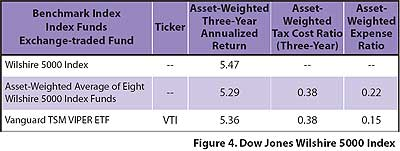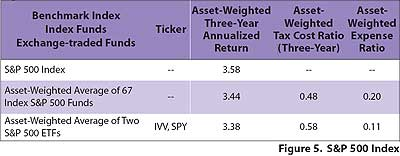# A Brave New World

July 01, 2005

Dow Jones Wilshire 5000

Our first comparison looked at ETFs and index funds that track the Dow Jones Wilshire 5000 Index (see Figure 4). This index offers a good example of the importance of asset weighting. The eight distinct index funds that track the Wilshire 5000 held a  total of \$36.9 billion in assets as of December 31, 2004. One of the funds (the Vanguard Total Stock Index Fund) held \$31.7 billion, or 86 percent of the total. Therefore, the average annualized return, tax cost ratio and expense ratio for that particular fund were weighted accordingly (at 86 percent). The data for the other seven funds were weighted according to their respective share of the total net assets.At year-end 2004, there was only one ETF that tracked the Wilshire 5000-the Vanguard Total Stock Market (TSM) VIPER (Vanguard Index Participation Equity Receipt). There were eight distinct index funds tracking the Wilshire 5000. As would be expected, the raw Dow Jones Wilshire 5000 Index had a higher three-year annualized return compared to the performance of both the index funds and the ETF. (Note: The benchmark for both the Vanguard Total Stock Market Fund and its VIPERs share class has since switched to the MSCI U.S. Broad Market Index. Also, it should be mentioned, that VIPERs are unique among ETFs in that they are one share class of a traditional mutual fund, and may therefore be expected to have different performance characteristics, especially regarding tax efficiency, when compared to stand-alone ETFs.)

The three-year annualized return of the ETF was 5.36 percent, or seven basis points higher than the asset-weighted average return of the eight index funds. The differential in expense ratio between the index funds and the ETF was seven basis points in favor of the ETF, thus the differential in performance is entirely  explained by the expense ratio, rather than tracking error.

The asset-weighted return of the index funds was 18 basis points lower than that of the raw index. The return of the ETF was 11 basis points lower than the raw index. In both cases, the differential in return is four basis points less than the expense ratio. This suggests that the funds and the ETF are delivering performance that partially offsets their expense ratios.

The asset-weighted average tax  cost ratio of the index funds was the same as the tax cost ratio of the ETF.

Standard & Poor's 500 Index

For many people, the Standard & Poor's 500 Index is the king of U.S. equity indexes. At year-end 2004, 67 index funds tracked it, with total assets of over \$203 billion (and recall that this is only the total number of distinct funds). In addition, there were two ETFs tracking the index, the SPDR and the iShares S&P 500 fund. Remarkably, these two ETFs had \$64 billion in assets at year-end 2004.

The average three-year asset-weighted return of the two ETFs was within 20 basis points of the return of the S&P 500 Index (see Figure 5). Given their average expense ratio of 11 basis points, the tracking error of the ETFs was only nine basis points. Tracking error, in this application, is defined as the differential in performance between the raw index and the fund or ETF that is not explained by the expense ratio.The asset-weighted three-year return of the 67 mutual funds that track the S&P 500 was 14 basis points lower than the raw index, or six basis points less than would be expected given the average expense ratio of 20 basis points. Moreover, the asset-weighted return of the index funds was six basis points higher than the asset-weighted return of the two ETFs. In spite of higher average expense ratios, the S&P 500 index funds outperformed the ETFs.

The tax-cost ratio of the index funds was 10 basis points better than the tax-cost ratio of the ETFs.